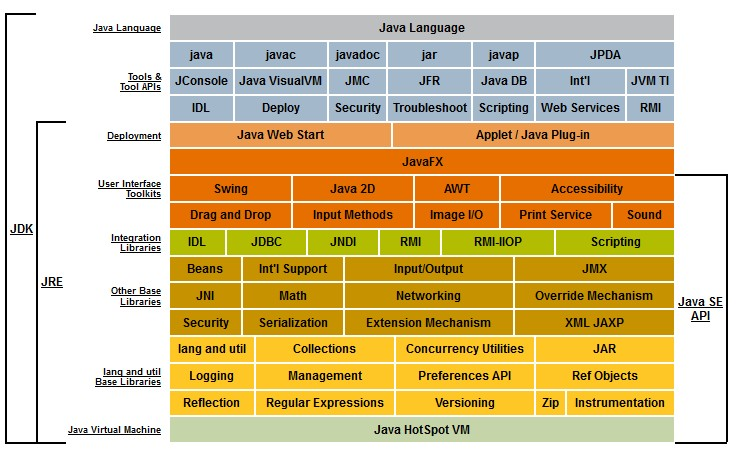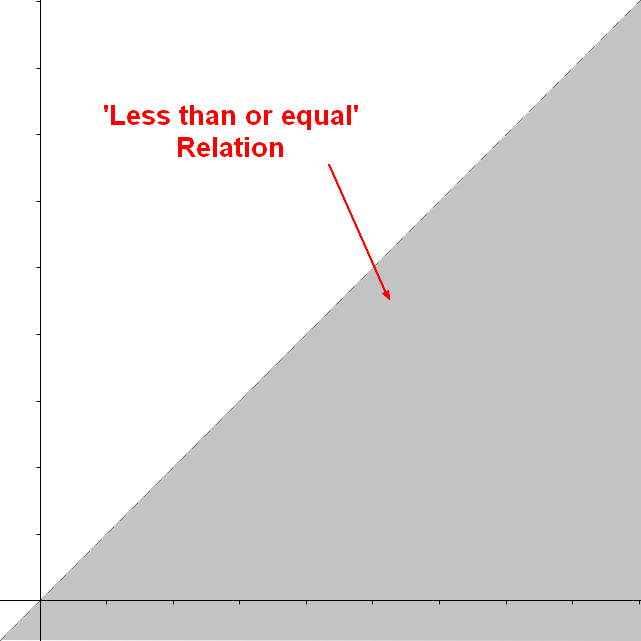# Sort - Greater Than operator

greater is a comparison operator that creates a binary relationship.

## Representation

### Set of tuples

The relation greater than or equal to (>=) on the set of integers {1, 2, 3} is the following set of tuple

<1, 1>,
<2, 1>,
<3, 1>,
<2, 2>,
<3, 2>,
<3, 3>



### Visual

The relationship greater than or equal between y and x (ie y >= x) can be represented in the set $R$ against $R^2$ as the below gray area:

Discover MoreFunction - Binary Function/Operation

A binary operation is an scalar operation with two arguments (arity of two) that produces one value. They are creating a binary relation. the addition operator, the multiplication operator ...Java - Greater than Operator

The greater than operator (>) The greater than operator (>) appliesonly to primitive types such as short, int, double, long, float, byte, and char. To compare objects, use a type parameter...Logical Data Modeling - Binary Relation

A binary relation is a relationship between two elements that is implemented via a binary function. Binary relations are used in many branches of mathematics to model concepts like: order relation...Logical Data Modeling - Reflexive relationship property

reflexive is a relationship property that indicates: that the relationship relates every element to itself. in other word, that the relation set representing the relationship contains every tuple:...Logical Data Modeling - Transitive Relationship Property

transitive is a relationship property that tells that the relationship follows the following rule: when the relation relates a to b and b to c, then the relation relates a to c. In mathematical notation,...Order - Relationship (Binary)

Every relationship in order is a binary relationship comparison such as greater than, is equal to. A comparison operator test the order relationship. dividesOrdinal Data - Comparison Operator (Order, Equality)

A comparison operator is a class of operators that model: a order or a equivalence relationship A comparison operator test the order relationship between two operands and returns a Boolean. The...Wat are Reserved Words (Keywords) in a language?

A reserved word is a special token in a language that cannot be used by the programmer because they are already used by the application and have a special meaning. In a URL, the backslash is used...
What is XML / HTML Character Entity encoding ?

An Entity in html is a string that represents a unicode character. unicode character Encoding text in HTML means to transform: the text characters into HTML entities. Example with the 0260Ephone....